# Ninety-Nine Problems in Kotlin

### Related tags

Kotlin Projects kotlin tree graph exercise

# Ninety-Nine Kotlin Problems## Introduction

This is an adaptation of Ninety-Nine Scala Problems by Phil Gold which itself is an adaptation of the Ninety-Nine Prolog Problems written by Werner Hett at the Berne University of Applied Sciences in Berne, Switzerland. Some problems have been altered to be more amenable to programming in Kotlin.

You might want to do these problems if you want to learn Kotlin, are interested in the problems described below, or both. The main reason to prefer this to using websites like hackerrank.com and codewars.com is that there is no vendor lock-in and no hidden agenda pursued by the website owner.

The suggested workflow is to solve a problem yourself and then compare solution to the one provided.
Solutions are available by clicking on the link at the beginning of the problem description. Your goal should be to find the most elegant solution to the given problems. Efficiency is important, but clarity is even more crucial. Some of the (easy) problems can be trivially solved using built-in functions. However, in these cases, you can learn more if you try to find your own solution.

The problems have different levels of difficulty. Those marked with a single asterisk `*` are easy. If you have successfully solved the preceding problems, you might be able to solve them within a few (say 15) minutes. Problems marked with two asterisks `**` are of intermediate difficulty and might take about 30-90 minutes to solve. Problems marked with three asterisks `***` are more difficult. You may need more time (i.e. a few hours or more) to find a good solution. Please note that levels of difficulty is just a guess and assumes you are somewhat familiar with the problem domain. It is perfectly ok if some of them take longer. Overall, the goal is to learn, not to finish "on time".

You might notice that there are less than 99 problems. This is because numbering of original problems was wrong. It was kept here for consistency with 99 problems in other programming languages.

The first 50 or so problems are easy. If this is boring for you, feel free to jump to Binary Trees.

All contributions are welcome (including alternative solutions for problems which already have a solution).

## Lists

### P01 (*) Find the last element of a list.

Example:

```> last(listOf(1, 1, 2, 3, 5, 8))
8```

### P02 (*) Find the last but one element of a list.

Example:

```> penultimate(listOf(1, 1, 2, 3, 5, 8))
5```

### P03 (*) Find the Nth element of a list.

By convention, the first element in the list is element `0`. Example:

```> nth(2, listOf(1, 1, 2, 3, 5, 8))
2```

### P04 (*) Find the number of elements of a list.

Example:

```> length(listOf(1, 1, 2, 3, 5, 8))
6```

### P05 (*) Reverse a list.

Example:

```> reverse(listOf(1, 1, 2, 3, 5, 8))
[8, 5, 3, 2, 1, 1]```

### P06 (*) Find out whether a list is a palindrome.

Example:

```> isPalindrome(listOf(1, 2, 3, 2, 1))
true```

### P07 (*) Flatten a nested list structure.

Example:

```> flatten(listOf(listOf(1, 1), 2, listOf(3, listOf(5, 8))))
[1, 1, 2, 3, 5, 8]```

### P08 (*) Eliminate consecutive duplicates of list elements.

If a list contains repeated elements, they should be replaced with a single copy of the element. The order of the elements should not be changed. Example:

```> compress("aaaabccaadeeee".toList())
[a, b, c, a, d, e]```

### P09 (*) Pack consecutive duplicates of list elements into sublists.

If a list contains repeated elements, they should be placed in separate sublists. Example:

```> pack("aaaabccaadeeee".toList())
[[a, a, a, a], [b], [c, c], [a, a], [d], [e, e, e, e]]```

### P10 (*) Run-length encoding of a list.

Use the result of problem P09 to implement the so-called run-length encoding data compression method. Consecutive duplicates of elements are encoded as tuples (N, E) where N is the number of duplicates of the element E. Example:

```> encode("aaaabccaadeeee".toList())
[(4, a), (1, b), (2, c), (2, a), (1, d), (4, e)]```

### P11 (*) Modified run-length encoding.

Modify the result of problem P10 in such a way that if an element has no duplicates it is simply copied into the result list. Only elements with duplicates are transferred as (N, E) terms. Example:

```> encodeModified("aaaabccaadeeee".toList())
[(4, a), b, (2, c), (2, a), d, (4, e)]```

### P12 (*) Decode a run-length encoded list.

Given a run-length code list generated as specified in the problem P10, construct its uncompressed version. Example:

```> decode(List((4, 'a), (1, 'b), (2, 'c), (2, 'a), (1, 'd), (4, 'e)))
[a, a, a, a, b, c, c, a, a, d, e, e, e, e]```

### P13 (*) Run-length encoding of a list (direct solution).

Implement the so-called run-length encoding data compression method directly. I.e. don't use other methods you've written (like P09's pack); do all the work directly. Example:

```> encodeDirect("aaaabccaadeeee".toList())
[(4, a), (1, b), (2, c), (2, a), (1, d), (4, e)]```

### P14 (*) Duplicate the elements of a list.

Example:

```> duplicate("abccd".toList())
[a, a, b, b, c, c, c, c, d, d]```

### P15 (*) Duplicate the elements of a list a given number of times.

Example:

```> duplicateN(3, "abccd".toList())
[a, a, a, b, b, b, c, c, c, c, c, c, d, d, d]```

### P16 (*) Drop every Nth element from a list.

Example:

```> drop(3, "abcdefghijk".toList())
[a, b, d, e, g, h, j, k]```

### P17 (*) Split a list into two parts.

The length of the first part is given. Use a `Pair` for your result. Example:

```> split(3, "abcdefghijk".toList())
([a, b, c], [d, e, f, g, h, i, j, k])```

### P18 (*) Extract a slice from a list.

Given two indices, I and K, the slice is the list containing the elements from and including the Ith element up to but not including the Kth element of the original list. Start counting the elements with 0. Example:

```> slice(3, 7, "abcdefghijk".toList())
[d, e, f, g]```

### P19 (*) Rotate a list N places to the left.

Examples:

```> rotate(3, "abcdefghijk".toList())
[d, e, f, g, h, i, j, k, a, b, c]

> rotate(-2, "abcdefghijk".toList())
[j, k, a, b, c, d, e, f, g, h, i]```

### P20 (*) Remove the Kth element from a list.

Return the list and the removed element in a Tuple. Elements are numbered from 0. Example:

```> removeAt(1, "abcd".toList())
([a, c, d], b)```

### P21 (*) Insert an element at a given position into a list.

Example:

```> insertAt('X', 1, "abcd".toList())
[a, X, b, c, d]```

### P22 (*) Create a list containing all integers within a given range.

Example:

```> range(4, 9)
[4, 5, 6, 7, 8, 9]```

### P23 (*) Extract a given number of randomly selected elements from a list.

Make sure there is a way to produce deterministic results. Example:

```> randomSelect(3, "abcdefgh".toList())
[c, h, f]```

### P24 (*) Lotto: Draw N different random numbers from the set 1..M.

Make sure there is a way to produce deterministic results. Example:

```> lotto(3, 49)
[32, 28, 8]```

### P25 (*) Generate a random permutation of the elements of a list.

Make sure there is a way to produce deterministic results. Hint: Use the solution of problem P23. Example:

```> randomPermute("abcdef".toList())
[d, b, e, f, a, c]```

### P26 (**) Generate the combinations of K distinct objects chosen from the N elements of a list.

In how many ways can a committee of 3 be chosen from a group of 12 people? There are `C(12,3) = 220` possibilities, where `C(N,K)` denotes binomial coefficient. For pure mathematicians, this result may be great. But we want to really generate all the possibilities. Example:

```> combinations(3, "abcde".toList())
[[c, b, a], [d, b, a], [e, b, a], [d, c, a], [e, c, a], [e, d, a], [d, c, b], [e, c, b], [e, d, b], [e, d, c]]```

### P27 (**) Group the elements of a set into disjoint subsets.

a) In how many ways can a group of 9 people work in 3 disjoint subgroups of 2, 3 and 4 persons? Write a function that generates all the possibilities. Example:

```> group3(listOf("Aldo", "Beat", "Carla", "David", "Evi", "Flip", "Gary", "Hugo", "Ida"))
[[["Ida", "Hugo", "Gary", "Flip"], ["Evi", "David", "Carla"], ["Beat", "Aldo"]], ...```

b) Generalize the above predicate in a way that we can specify a list of group sizes and the predicate will return a list of groups. Example:

```> group(listOf(2, 2, 5), listOf("Aldo", "Beat", "Carla", "David", "Evi", "Flip", "Gary", "Hugo", "Ida"))
[[["Ida", "Hugo", "Gary", "Flip", "Evi"], ["David", "Carla"], ["Beat", "Aldo"]], ...```

Note that we do not want permutations of the group members, i.e. `[[Aldo, Beat], ...]]` is the same solution as `[[Beat, Aldo], ...]`. However, `[[Aldo, Beat], [Carla, David], ...]` and `[[Carla, David], [Aldo, Beat], ...]` are considered to be different solutions.

You may find more about this combinatorial problem in a good book on discrete mathematics under the term multinomial coefficients.

### P28 (*) Sorting a list of lists according to length of sublists.

a) We suppose that a list contains elements that are lists themselves. The objective is to sort elements of the list according to their length. E.g. short lists first, longer lists later, or vice versa. Example:

```> lengthSort(listOf("abc".toList(), "de".toList(), "fgh".toList(), "de".toList(), "ijkl".toList(), "mn".toList(), "o".toList()))
[[o], [d, e], [d, e], [m, n], [a, b, c], [f, g, h], [i, j, k, l]]```

b) Again, we suppose that a list contains elements that are lists themselves. But this time the objective is to sort elements according to their length frequency; i.e. lists with rare lengths are placed first, others with more frequent lengths come later. Example:

```> lengthFreqSort(listOf("abc".toList(), "de".toList(), "fgh".toList(), "de".toList(), "ijkl".toList(), "mn".toList(), "o".toList()))
[[i, j, k, l], [o], [a, b, c], [f, g, h], [d, e], [d, e], [m, n]]```

Note that in the above example, the first two lists in the result have length 4 and 1 and both lengths appear just once. The third and fourth lists have length 3 and there are two list of this length. Finally, the last three lists have length 2. This is the most frequent length.

## Arithmetic

### P31 (*) Determine whether a given integer number is prime.

```> 7.isPrime()
true```

### P32 (*) Determine the greatest common divisor of two positive integer numbers.

```> gcd(36, 63)
9```

### P33 (*) Determine whether two positive integer numbers are coprime.

Two numbers are coprime if their greatest common divisor equals 1.

```> 35.isCoprimeTo(64)
true```

### P34 (*) Calculate Euler's totient function phi(m).

Euler's so-called totient function phi(m) is defined as the number of positive integers r (1 <= r <= m) that are coprime to m.

```> 10.totient()
4```

### P35 (*) Determine prime factors of a given positive integer.

Construct a list containing prime factors in ascending order.

```> 315.primeFactors()
[3, 3, 5, 7]```

### P36 (*) Determine the prime factors of a given positive integer (2).

Construct a list containing prime factors and their multiplicity.

```> 315.primeFactorMultiplicity()
[(3, 2), (5, 1), (7, 1)]```

### P37 (*) Calculate Euler's totient function phi(m) (improved).

See problem P34 for the definition of Euler's totient function. If the list of the prime factors of a number `m` is known in the form of problem P36, then the function `phi(m)` can be efficiently calculated as follows: Let `[[p1, m1], [p2, m2], [p3, m3], ...]` be the list of prime factors (and their multiplicities) of a given number `m`. Then `phi(m)` can be calculated with the following formula: `phi(m) = (p1-1)*p1^(m1-1) * (p2-1)*p2^(m2-1) * (p3-1)*p3^(m3-1) * ...`

### P38 (*) Compare the two methods of calculating Euler's totient function.

Omitted. The assumption is that you already did the comparison, e.g. as unit test assertions.

### P39 (*) A list of prime numbers.

Given a range of integers by its lower and upper limit, construct a list of all prime numbers in that range.

```> listPrimesInRange(7..31)
[7, 11, 13, 17, 19, 23, 29, 31]```

### P40 (*) Goldbach's conjecture.

Goldbach's conjecture says that every positive even number greater than 2 is the sum of two prime numbers. E.g. `28 = 5 + 23`. It is one of the most famous facts in number theory that has not been proved to be correct in the general case. It has been numerically confirmed up to very large numbers (much larger than Kotlin's Int can represent). Write a function to find the two prime numbers that sum up to a given even integer.

```> 28.goldbach()
(5, 23)```

### P41 (*) A list of Goldbach compositions.

Given a range of integers by its lower and upper limit, print a list of all even numbers and their Goldbach composition.

```> printGoldbachList(9..20)
10 = 3 + 7
12 = 5 + 7
14 = 3 + 11
16 = 3 + 13
18 = 5 + 13
20 = 3 + 17```

In most cases, if an even number is written as the sum of two prime numbers, one of them is very small. Very rarely, the primes are both bigger than, say, 50. Example (minimum value of 50 for the primes):

```> printGoldbachListLimited(2..3000, 50)
992 = 73 + 919
1382 = 61 + 1321
1856 = 67 + 1789
...```

## Logic and Codes

### P46 (*) Truth tables for logical expressions.

Define functions `and_`, `or_`, `nand_`, `nor_`, `xor_`, `impl_`, and `equ_` (for logical equivalence) which return `true` or `false` according to the result of their respective operations.

```> true.and_(true)
true
> true.xor_(true)
false```

Write a function called `printTruthTable` which prints the truth table of a given logical expression.

```> printTruthTable{ a, b -> a.and_(a.or_(b.not_())) }
a	b	result
true	true	true
true	false	true
false	true	false
false	false	false```

### P47 (*) Truth tables for logical expressions (2).

For Scala the task was to use implicit conversion. This is much simpler in Kotlin so the task omitted assuming it was done in the previous problem.

### P48 (*) Truth tables for logical expressions (3).

Generalize problem 46 in such a way that the logical expression may contain any number of logical variables. Example:

```> true.xor_(true, false, true)
true```

### P49 (*) Gray code.

An n-bit Gray code is a sequence of n-bit strings constructed according to certain rules. Find out the construction rules and write a function to generate Gray codes. For example:

```> grayCodes(bits = 1)
[0, 1]
> grayCodes(bits = 2)
[00, 01, 11, 10]
> grayCodes(bits = 3)
[000, 001, 011, 010, 110, 111, 101, 100]```

### P50 (**) Huffman code.

If you are not familiar with Huffman coding, consult internet (or a good book).

a) Given characters with their frequencies, e.g. `{a=25, b=21, c=18, d=14, e=9, f=7, g=6}`. Our objective is to construct a `Map`, where key is character and value is the Huffman code for it.

```> createEncoding(linkedMapOf(Pair('a', 25), Pair('b', 21), Pair('c', 18), Pair('d', 14), Pair('e', 9), Pair('f', 7), Pair('g', 6)))
{a=10, b=00, c=111, d=110, e=010, f=0111, g=0110}```

b) Write `encode` and `decode` functions for conversion between `String` and encoded `String` with zeroes and ones. For example:

```"this is a sentence".encode(encoding)
"00110000101011100101011101001110101111011001111011000111"

"00110000101011100101011101001110101111011001111011000111".decode(encoding)
"this is a sentence"```

## Binary Trees

A binary tree is either empty or it is composed of a root element and two successors, which are binary trees themselves.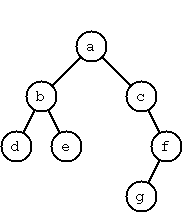We will use the following classes to represent binary trees (see Tree.kt). An `End` is equivalent to an empty tree. A `Node` has a value, and two child trees. The `toString()` functions are relatively arbitrary and were written to produce minimal readable output. Note the usage of variance annotation `out T` which makes classes covariant; it will be able to hold subtypes of whatever type it's created for. `End` is declared as value because data classes must have at least one constructor parameter. `End` has type parameter of `Nothing` which is a subtype of every other type.

```interface Tree<out T>

data class Node<out T>(val value: T, val left: Tree<T> = End, val right: Tree<T> = End) : Tree<T> {
override fun toString(): String {
val children = if (left == End && right == End) "" else " \$left \$right"
return "T(\$value\$children)"
}
}

val End = object : Tree<Nothing>{
override fun toString() = "."
}```

The example of tree above can be written as:

```Node('a',
Node('b',
Node('d'),
Node('e')),
Node('c', End,
Node('f', Node('g'),
End)))```

A tree with only a root node would be `Node('a')` and an empty tree would be `End`.

### P54 Omitted; our tree representation will only allow well-formed trees.

Score one for static typing.

### P55 (*) Construct completely balanced binary trees.

In a completely balanced binary tree, the following property holds for every node. The number of nodes in its left subtree and the number of nodes in its right subtree are almost equal, which means their difference is not greater than one. Define an object named Tree. Write a function `balancedTrees` to construct completely balanced binary trees for a given number of nodes. The function should generate all solutions. The function should take as parameters the number of nodes and a single value to put in all of them.

```> balancedTrees(4, "x")
[T(x T(x) T(x . T(x))), T(x T(x . T(x)) T(x)), T(x T(x) T(x T(x) .)), T(x T(x T(x) .) T(x))]```

### P56 (*) Symmetric binary trees.

Let us call a binary tree symmetric if you can draw a vertical line through the root node and then the right subtree is the mirror image of the left subtree. Add an `isSymmetric` method to the `Tree` to check whether a given binary tree is symmetric. Hint: Write `isMirrorOf` method first to check whether one tree is the mirror image of another. We are only interested in the structure, not in the contents of the nodes.

```> Node("a", Node("b"), Node("c")).isSymmetric()
true```

### P57 (*) Binary search trees (dictionaries).

Write a function to add an element to a binary search tree.

```> End.add(2)
T(2)
T(2 . T(3))
T(2 T(0) T(3))```

Note that definition of `add` should have `T : Comparable<T>` type constraint to allows us to use the `<` operator on the values in the tree.

Use that function to construct a binary tree from a list of integers.

```> listOf(3, 2, 5, 7, 1).toTree()
T(3 T(2 T(1) .) T(5 . T(7)))```

Finally, use `isSymmetric()` from P56 to check conversion to tree.

```> listOf(5, 3, 18, 1, 4, 12, 21).toTree().isSymmetric()
true
> listOf(3, 2, 5, 7, 4).toTree().isSymmetric()
false```

Apply the generate-and-test paradigm to construct all symmetric, completely balanced binary trees with a given number of nodes.

```> symmetricBalancedTrees(5, "x")
[T(x T(x . T(x)) T(x T(x) .)), T(x T(x T(x) .) T(x . T(x)))]```

### P59 (**) Construct height-balanced binary trees.

In a height-balanced binary tree, the following property holds for every node: The height of its left subtree and the height of its right subtree are almost equal, which means their difference is not greater than one. Write a method `heightBalancedTrees` to construct height-balanced binary trees for a given height with a supplied value for the nodes. The function should generate all solutions.

```> heightBalancedTrees(3, "x")
[T(x T(x T(x) T(x)) T(x T(x) T(x))), T(x T(x T(x) T(x)) T(x T(x) .)), ...]```

### P60 (**) Construct height-balanced binary trees with a given number of nodes.

Consider a height-balanced binary tree of height `H`. The maximum number of nodes it can contain is `MaxN = 2**H - 1`. However, what is the minimum number `MinN`? This question is more difficult. Try to find a recursive statement and turn it into a function `minNodeAmountInHBTree` that takes a height and returns `MinN`.

```> minNodeAmountInHBTree(height = 3)
4```

On the other hand, we might ask: what is the maximum height `H` a height-balanced binary tree with `N` nodes can have? Write a `maxHeightOfHBTree` function.

```> maxHeightOfHBTree(nodeAmount = 4)
3```

Now, we can attack the main problem: construct all the height-balanced binary trees with a given number of nodes.

```> allHBTreesWithNodeAmount(4, "x")
[T(x T(x T(x) .) T(x)), T(x T(x . T(x)) T(x)), ...]```

Find out how many height-balanced trees exist for `N = 15`.

### P61 (*) Leaves and internal nodes of a binary tree.

A leaf is a node with no successors. Write a method `leafCount` to count them.

```> Node("x", Node("x"), End).leafCount()
1```

Write a method `leafValues` to collect leaf values into a list.

```> Node("a", Node("b"), Node("c", Node("d"), Node("e"))).leafValues()
[b, d, e]```

An internal node of a binary tree has either one or two non-empty successors. Write a method `internalValues` to collect their values into a list.

```> Node("a", Node("b"), Node("c", Node("d"), Node("e"))).internalValues()
[a, c]```

### P62 (*) Collect nodes at a given level in a list.

A node of a binary tree is at level `N` if the path from the root to the node has length `N-1`. The root node is at level 1. Write a method `valuesAtLevel` to collect all node values at a given level into a list.

```> Node('a', Node('b'), Node('c', Node('d'), Node('e'))).valuesAtLevel(2)
[b, c]```

Using `valuesAtLevel` it is easy to construct a method to create the level-order sequence of the nodes. However, there are more efficient ways to do that.

### P63 (*) Construct a complete binary tree.

A complete binary tree with height `H` is defined as follows: The levels `1,2,3,...,H-1` contain the maximum number of nodes, i.e `2(i-1)` nodes at the level `i` (note that we start counting the levels from 1 at the root). At level `H`, which may contain less than the maximum possible number of nodes, all the nodes are "left-adjusted". This means that in a level-order tree traversal all internal nodes come first, the leaves come second, and empty successors (the `End`s which are not really nodes) come last. Particularly, complete binary trees are used as data structures (or addressing schemes) for heaps.

We can assign an address number to each node in a complete binary tree by enumerating the nodes in level order, starting at the root with number 1. In doing so, we realize that for every node `X` with address `A` the following property holds: The address of `X`'s left and right children are `2*A` and `2*A+1` (assuming the children exist). This fact can be used to elegantly construct a complete binary tree structure.

Write a method `completeBinaryTree` that takes as parameters the number of nodes and the value to put in each node.

```> completeBinaryTree(6, "x")
T(x T(x T(x) T(x)) T(x T(x) .))```

### P64 (**) Layout a binary tree (1).

As a preparation for drawing a tree, a layout algorithm is required to determine the position of each node in a rectangular grid. Several layout methods are conceivable, one of them is shown in the illustration below. This tree can be constructed with `"nkmcahgeupsq".toList().toTree()`.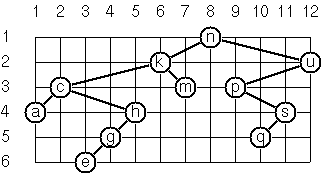In this layout strategy, the position of a node `v` is obtained by the following two rules:

• `x(v)` is equal to the position of the node `v` in the in-order sequence
• `y(v)` is equal to the depth of the node `v` in the tree In order to store the position of the nodes, we add a new data classes with the additional information.
```data class Point(val x: Int, val y: Int)

data class Positioned<out T>(val value: T, val point: Point) {
constructor (value: T, x: Int, y: Int) : this(value, Point(x, y))

override fun toString(): String =
"[" + point.x.toString() + "," + point.y.toString() + "] " + value.toString()
}```

Write a method `layout` that turns a tree of normal `Node`s into a tree with positions `Tree<Positioned<T>>`.

```> Node("a", Node("b", End, Node("c")), Node("d")).layout()
T([3,1] a T([1,2] b . T([2,3] c)) T([4,2] d))```

### P65 (**) Layout a binary tree (2).

An alternative layout method is depicted in the illustration below (note that it is not the same tree as in the previous problem). This tree can be constructed with `"nkmcaedgupq".toList().toTree()`.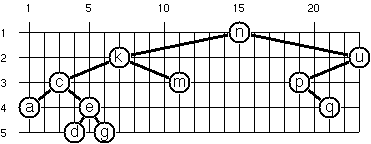Find out the rules and write the corresponding method. Hint: On a given level, the horizontal distance between neighboring nodes is constant. Use the same conventions as in the problem P64.

```> Node("a", Node("b", End, Node("c")), Node("d")).layout2()
T[3,1]('a T[1,2]('b . T[2,3]('c . .)) T[5,2]('d . .))```

### P66 (***) Layout a binary tree (3).

Yet another layout strategy is shown in the illustration below. This tree can be constructed with `"nkmcaedgupq".toList().toTree()`.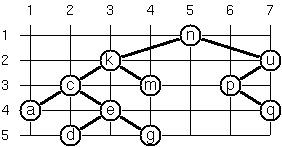The method yields a very compact layout while maintaining a certain symmetry in every node. Find out the rules and write the corresponding method. Hint: Consider the horizontal distance between a node and its successor nodes. How tight can you pack together two subtrees to construct the combined binary tree? Use the same conventions as in problem P64 and P65. Note: This is a difficult problem. Don't give up too early!

```> Node('a', Node('b', End, Node('c')), Node('d')).layoutBinaryTree3()
T[2,1]('a T[1,2]('b . T[2,3]('c . .)) T[3,2]('d . .))```

Which layout do you like most?

### P67 (**) A string representation of binary trees.

Binary trees can be represented as strings of the following type: `a(b(d,e),c(,f(g,)))`.Write a method which generates this string representation given tree as `Node`s and `End`s. And a method which does this inverse, i.e. given the string representation, construct the tree in the usual form.

```> Node("a", Node("b", Node("d"), Node("e")), Node("c", End, Node("f", Node("g"), End))).convertToString()
a(b(d,e),c(,f(g,)))
> "a(b(d,e),c(,f(g,)))".convertToTree()
T(a T(b T(d) T(e)) T(c . T(f T(g) .)))```

### P68 (**) Preorder and inorder sequences of binary trees.

a) Write methods `preorder` and `inorder` that construct the pre-order and in-order sequence of a given binary tree, respectively. The results should be lists, e.g. `["a","b","d","e","c","f","g"]` for the preorder sequence of the example in problem P67.

```> "a(b(d,e),c(,f(g,)))".convertToTree().preorder()
[a, b, d, e, c, f, g]
> "a(b(d,e),c(,f(g,)))".convertToTree().inorder()
[d, b, e, a, c, g, f]```

b) If both the preorder sequence and the in-order sequence of the nodes of a binary tree are given, then the tree is determined unambiguously. Write a method `createTree` that does the job.

```> createTree(preorder = listOf("a", "b", "d", "e", "c", "f", "g"), inorder = listOf("d", "b", "e", "a", "c", "g", "f"))
a(b(d,e),c(,f(g,)))```

What happens if the same character appears in more than one node? Try, for instance:

`createTree(preorder = listOf("a", "b", "a"), inorder = listOf("b", "a", "a"))`

### P69 (*) Dot-string representation of binary trees.

Binary tree in which leaves contain only single characters can be represented by the preorder sequence of its nodes in which dots `.` are inserted where an empty subtree `End` is encountered during tree traversal. For example, the tree shown in problem P67 is represented as `abd..e..c.fg...`.

First, try to establish a syntax (BNF or syntax diagrams) and then write two methods, `toDotString` and `fromDotString`, which do the conversion in both directions.

```> "a(b(d,e),c(,f(g,)))".convertToTree().toDotString()
abd..e..c.fg...
> "abd..e..c.fg...".fromDotString()
a(b(d,e),c(,f(g,)))```

## Multiway Trees

A multiway tree is composed of a root element and a (possibly empty) set of successors which are multiway trees themselves. A multiway tree is never empty. The set of successor trees is sometimes called a forest.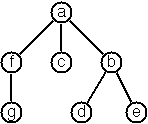The code to represent multiway-trees is somewhat simpler than the code for binary trees, partly because we don't separate classes for nodes and terminators, and partly because we don't need the restriction for the value type to be ordered.

```data class MTree<out T>(val value: T, val children: List<MTree<T>> = emptyList()) {

constructor(value: T, vararg children: MTree<T>): this(value, children.toList())

override fun toString(): String =
if (children.isEmpty()) value.toString()
else value.toString() + " {" + children.joinToString(", "){ it.toString() } + "}"
} ```

The example tree is, thus:

```MTree("a",
MTree("f",
MTree("g")),
MTree("c"),
MTree("b",
MTree("d"), MTree("e"))
))
```

The starting code for this section is in MTree.kt.

### P70A (*) Count the nodes of a multiway tree.

Write a method `nodeCount` which counts the nodes of a given multiway tree.

```> MTree("a", MTree("f")).nodeCount()
2```

### P70B (*) Tree construction from a node string.

Suppose that the nodes of a multiway tree contain single characters. In the depth-first order sequence of its nodes, a special character `^` has been inserted whenever, during the tree traversal, the move is a backtrack to the previous level. By this rule, the tree in the following figure is represented as: `afg^^c^bd^e^^^`.Define the syntax of the string and write a function `convertToMTree` to construct an `MTree` from a `String`. Write the reverse `convertToString` function.

```> MTree('a', MTree('f', MTree('g')), MTree('c'), MTree('b', MTree('d'), MTree('e'))).toString()
afg^^c^bd^e^^^```

### P71 (*) Determine the internal path length of a tree.

We define the internal path length of a multiway tree as the total sum of the path lengths from the root to all nodes of the tree. By this definition, the tree in the figure of problem P70B has an internal path length of 9. Write a method `internalPathLength` to return that sum.

```> "afg^^c^bd^e^^^".convertToMTree().internalPathLength()
9```

### P72 (*) Construct the postorder sequence of the tree nodes.

Write a method postorder which constructs the postorder sequence of the node values of a multiway tree. The result should be a `List`.

```> "afg^^c^bd^e^^^".convertToMTree().postorder()
[g, f, c, d, e, b, a]```

### P73 (**) Lisp-like tree representation.

Lisp is a prominent functional programming language. In Lisp almost everything is a list. Our example tree would be represented in Lisp as `(a (f g) c (b d e))`. The following pictures give some more examples.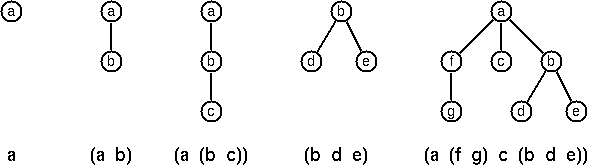Note that in the Lisp notation a node with successors (children) in the tree is always the first element in a list, followed by its children. The "lispy" representation of a multiway tree is a sequence of atoms and parentheses `(` and `)`, with the atoms separated by spaces.

a) Write a method `toLispString` which constructs a "lispy" `String` from an `MTree`.

```> MTree("a", MTree("b", MTree("c"))).toLispString()
(a (b c))```

b) As a second, even more interesting, exercise try to write a method that takes a "lispy" string and turns it into a multiway tree.

```> "(a (f g) c (b d e))".fromLispString()
a {f {g}, c, b {d, e}}```

## Graphs

(Warning! The introductory text below is quite long. If you are familiar with graphs, you might just look at source code in Graph.kt.)

A graph is defined as a set of nodes and a set of edges, where each edge is a pair of nodes.

The class to represent a graph is mutable, which isn't in keeping with pure functional programming, but a pure functional data structure would make things much, much more complicated. Pure functional graphs with cycles require laziness; Kotlin can probably handle it, but I think that would add too much of a barrier to the following questions.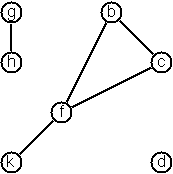Our graphs use an incidence list internally. Each has a list of nodes and a list of edges. Each node also has a list of edges that connect it to other nodes. In a directed graph, nodes, that are the target of arcs, do not have references to those arcs in their adjacency list.

```class Graph<T, U> {
val nodes: MutableMap<T, Node<T, U>> = HashMap()
val edges: MutableList<Edge<T, U>> = ArrayList()

fun addNode(value: T): Node<T, U> {
val node = Node<T, U>(value)
nodes.put(value, node)
return node
}

fun addUndirectedEdge(n1: T, n2: T, label: U?) {
if (!nodes.contains(n1) || !nodes.contains(n2)) {
throw IllegalStateException("Expected '\$n1' and '\$n2' nodes to exist in graph")
}
val edge = UndirectedEdge(nodes[n1]!!, nodes[n2]!!, label)
if (edges.all{ !it.equivalentTo(edge) }) {
}
}

fun addDirectedEdge(source: T, dest: T, label: U?) {
val edge = DirectedEdge(nodes[source]!!, nodes[dest]!!, label)
if (!edges.contains(edge)) {
}
}

data class Node<T, U>(val value: T) {
val edges: MutableList<Edge<T, U>> = ArrayList()
fun neighbors(): List<Node<T, U>> = edges.map{ edge -> edge.target(this)!! }
override fun toString() = value.toString()
}

interface Edge<T, U> {
val n1: Node<T, U>
val n2: Node<T, U>
val label: U?
fun target(node: Node<T, U>): Node<T, U>?
fun equivalentTo(other: Edge<T, U>) =
(n1 == other.n1 && n2 == other.n2) || (n1 == other.n2 && n2 == other.n1)
}

data class UndirectedEdge<T, U>(override val n1: Node<T, U>, override val n2: Node<T, U>, override val label: U?) : Edge<T, U> {
override fun target(node: Node<T, U>) = if (n1 == node) n2 else if (n2 == node) n1 else null
override fun toString() = n1.toString() + "-" + n2 + (if (label == null) "" else "/" + label.toString())
}

data class DirectedEdge<T, U>(override val n1: Node<T, U>, override val n2: Node<T, U>, override val label: U?) : Edge<T, U> {
override fun target(node: Node<T, U>) = if (n1 == node) n2 else null
override fun toString() = n1.toString() + ">" + n2 + (if (label == null) "" else "/" + label.toString())
}
}
```

There are a few ways to create a graph from primitives. The graph-term form lists the nodes and edges separately:

```Graph.terms(TermForm(
nodes = listOf("b", "c", "d", "f", "g", "h", "k"),
edges = listOf(Term("b", "c"), Term("b", "f"), Term("c", "f"), Term("f", "k"), Term("g", "h"))))```

The adjacency-list form associates each node with its adjacent nodes. In an undirected graph, care must be taken to ensure that all links are symmetric, i.e. if `b` is adjacent to `c`, `c` must also be adjacent to `b`.

```Graph.adjacent(AdjacencyList(
Entry("d"),

The representations we introduced so far are bound to our implementation and therefore well suited for automated processing, but their syntax is not very user-friendly. Typing the terms by hand is cumbersome and error-prone. We can define a more compact and "human-friendly" notation as follows: A graph is represented by a string of terms of the type `X` or `Y-Z` separated by commas. The standalone terms stand for isolated nodes, the `Y-Z` terms describe edges. If an `X` appears as an endpoint of an edge, it is automatically defined as a node. Our example could be written as:

``````[b-c, f-c, g-h, f-b, k-f, h-g, d]
``````

We call this the human-friendly form. As the example shows, the list does not have to be sorted and may even contain the same edge multiple times. Notice the isolated node `d`.

Directed graph is a graph where edges have direction. To represent a directed graph, the forms discussed above are slightly modified. The example graph is represented as follows: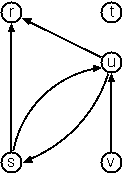In graph-term form:

```Graph.directedTerms(TermForm(
listOf("r", "s", "t", "u", "v"),
listOf(Term("s", "r"), Term("s", "u"), Term("u", "r"), Term("u", "s"), Term("v", "u"))))```

In adjacency-list form (note that the adjacency-list form is the same for graphs and digraphs):

```Graph.directedAdjacent(AdjacencyList(
Entry("r"),
Entry("t"),

Human-friendly form:

``````[s>r, s>u, u>r, u>s, v>u, t]
``````

Finally, graphs with additional information attached to edges are called labeled graphs.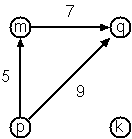Graph-term form:

```Graph.labeledTerms(TermForm(
listOf("k", "m", "p", "q"),
listOf(Term("m", "q", 7), Term("p", "m", 5), Term("p", "q", 9))))```

```Graph.labeledDirectedAdjacent(AdjacencyList(
Entry("k"),
Entry("q")))```

Human-friendly form:

``````[m-q/7, p-m/5, p-q/9, k]
``````

The notation for labeled graphs can also be used for so-called multi-graphs, where more than one edge is allowed between two given nodes.

### P80 (*) Conversions.

Write `String.toGraph()` and `String.toLabeledGraph()` functions to create graphs from strings (you can detect if graph is labeled or unlabeled based on input string format). Write functions `toTermForm` and `toAdjacencyList` to generate the graph-term and adjacency-list forms of a `Graph`.

```> "[b-c, b-f, c-f, f-k, g-h, d]".toGraph().toTermForm()
TermForm(nodes=[f, g, d, b, c, k, h], edges=[Term(b, c), Term(b, f), Term(c, f), Term(f, k), Term(g, h)])

### P81 (***) Path between nodes.

a) Write method `findAllPaths` to find acyclic paths from one node to another in a graph. The method should return all paths.

```> "[p>q/9, m>q/7, k, p>m/5]".toLabeledGraph().findAllPaths("p", "q")
[[p, q], [p, m, q]]
> "[p>q/9, m>q/7, k, p>m/5]".toLabeledGraph().findAllPaths("p", "k")
[]```

b) Write method `findShortestPath` to find shortest path between two nodes. Hint: use Dijkstra or A* algorithm.

```"[a-b/1, b-c/1, a-c/3]".toLabeledGraph().findShortestPath("a", "c")
[a, b, c]```

### P82 (*) Cycles from a node.

Write a method named `findCycles` to find closed paths (cycles) starting at a given node in a graph. The method should return all cycles. (Note that single edge doesn't count as a cycle.)

```> "[a-b]".toGraph().findCycles("a")
[]
> "[b-c, b-f, c-f, f-k, g-h, d]".toGraph().findCycles("f")
[[f, c, b, f], [f, b, c, f]]```

### P83 (**) Spanning trees.

Write a method `spanningTrees` to construct all spanning trees of a given graph.

When you have a correct solution for the `spanningTrees` method, use it to define two other useful methods: `Graph.isTree` and `Graph.isConnected`.

```> "[a-b, b-c, a-c]".toGraph().spanningTrees()
[[a-b, b-c], [a-b, c-a], [b-c, c-a]]
> "[a-b, b-c, a-c]".toGraph().isTree()
false
> "[a-b, b-c, a-c]".toGraph().isConnected()
true```

Find out how many spanning trees there are for the graph depicted below.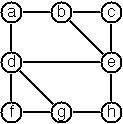`"[a-b, a-d, b-c, b-e, c-e, d-e, d-f, d-g, e-h, f-g, g-h]".toGraph()`

### P84 (**) Minimum spanning tree.

Write a method `minSpanningTree` to construct the minimum spanning tree of a given labeled graph. Hint: Use Prim's Algorithm.

```> "[a-b/1, b-c/2, a-c/3]".toLabeledGraph().minSpanningTree()
[a-b/1, b-c/2]```

Find minimum spanning tree for the graph below: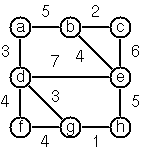`"[a-b/5, a-d/3, b-c/2, b-e/4, c-e/6, d-e/7, d-f/4, d-g/3, e-h/5, f-g/4, g-h/1]".toLabeledGraph()`

### P85 (**) Graph isomorphism.

Two graphs `G1(N1,E1)` and `G2(N2,E2)` are isomorphic if there is a bijection `f: N1 → N2` such that for any nodes `X`,`Y` of `N1`, `X` and `Y` are adjacent if and only if `f(X)` and `f(Y)` are adjacent.

Write a method that determines whether two graphs are isomorphic.

```> "[a-b]".toGraph().isIsomorphicTo("[5-7]".toGraph())
true
> "[a-b, b-c]".toGraph().isIsomorphicTo("[1-2, 3]".toGraph())
false
> "[a-b, b-c, c-d, d-a]".toGraph().isIsomorphicTo("[1-2, 2-3, 3-4, 4-1]".toGraph()
true```

### P86 (**) Node degree and graph coloration.

a) Write a method `Node.degree` that determines the degree of a given node in an undirected graph.

```> "[a-b, b-c, a-c, a-d]".toGraph().nodes["a"].degree()
3```

b) Use Welsh-Powell's algorithm to paint the nodes of an undirected graph in such a way that adjacent nodes have different colors. Write a method `colorNodes` that returns a list of tuples, each of which contains a node and an integer representing its color.

```> "[a-b, b-c, a-c, a-d]".toGraph().colorNodes()
[(a,1), (b,2), (c,3), (d,2)]```

### P87 (**) Depth-first order graph traversal.

a) Write a method that generates a depth-first order graph traversal sequence. The starting point should be specified, and the output should be a list of nodes that are reachable from this starting point (in depth-first order).

```> "[a-b, b-c, c-d, d-e]".toGraph().nodesByDepthFrom("c")
[c, b, a, d, e]```

b) Write similar method for breadth-first graph traversal.

```> "[a-b, b-c, c-d, d-e]".toGraph().nodesByBreadthFrom("c")
[c, b, d, a, e]```

### P88 (*) Connected components.

Write a function that splits a graph into its connected components.

```> "[a-b, c-d]".toGraph().components()
[[a-b], [c-d]]```

### P89 (**) Bipartite graphs.

Write a function that determines whether a given graph is bipartite.

```> "[a-b, b-c]".toGraph().isBipartite()
true
> "[a-b, b-c, c-a]".toGraph().isBipartite()
false
> "[a-b, b-c, d]".toGraph().isBipartite()
true
> "[a-b, b-c, d, e-f, f-g, g-e, h]".toGraph().isBipartite()
false
> "[a>b, c>a, d>b]".toGraph().isBipartite()
true```

## Miscellaneous

### P90 (**) Eight queens.

This is a classical problem in computer science. The objective is to place eight queens on a chessboard so that no two queens are attacking each other, i.e. no two queens are in the same row, column or diagonal.

Hint: it might be easier to represent positions of the queens as a list of numbers `1..N`. For example, `listOf(4, 2, 7, 3, 6, 8, 5, 1)` meaning that queen in the first column is in row 4, the queen in the second column is in row 2, etc. Otherwise, feel free to use a data class for queen position.

### P91 (**) Knight's tour.

This is another classical problem in computer science. How can a knight jump on an `N×N` chessboard in such a way that it visits every square exactly once?

Write a function `knightsTours(N, (X, Y))` to list all knight tours that be made from `(X, Y)` on a `N×N` chessboard. Hints: It might help to represent squares by pairs of their coordinates of the form `Pair(X, Y)`, where `X` and `Y` are integers between `0` and `N-1`. Alternatively, define a `Point` data class for this purpose.

Can you find only "closed tours", where the knight can jump from its final position back to its starting position? Can you make a lazy list that only calculates the tours as needed?

Several years ago I met a mathematician who was intrigued by a problem for which he didn't know a solution. His name was Von Koch, and I don't know whether the problem has been solved since. (The "I" here refers to the author of the Prolog problems.)

Anyway the puzzle goes like this: Given a tree with `N` nodes (and hence `N-1` edges), find a way to enumerate the nodes from `1` to `N` and, accordingly, the edges from `1` to `N-1` in such a way, that for each edge `K` the difference of its node numbers is equal to `K`. The conjecture is that this is always possible.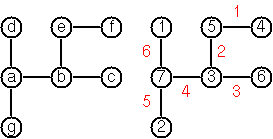For small trees the problem is easy to solve by hand. However, for larger trees, and 14 is already very large, it is extremely difficult to find a solution. And remember, we don't know for sure whether there is always a solution!

Write a function that calculates a numbering scheme for a given tree. What is the solution for the larger tree pictured below?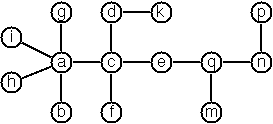### P93 (***) An arithmetic puzzle.

Given a list of integer numbers, find a correct way of inserting arithmetic operators `+-*/()` such that the result is a correct equation. Example: With the list of numbers `2, 3, 5, 7, 11` we can form the equations `2 - 3 + 5 + 7 = 11`, `2 = (3 * 5 + 7) / 11` and others.

### P94 (***) Regular graphs with N nodes.

In a K-regular graph all nodes have a degree of `K`, i.e. the number of edges incident in each node is `K`. Write a function to find all non-isomorphic 3-regular graphs with 6 nodes.

### P95 (**) English number words.

On financial documents, like checks, numbers must sometimes be written in full words. For example, `175` will be written as `one hundred seventy five`. Write a function `Int.toWords()` to convert (non-negative) integer numbers to words.

### P96 (**) Conway's Game of Life.

The Game of Life, is a cellular automaton devised by the British mathematician John Horton Conway in 1970.

The game is represented by a 2-dimensional grid populated with cells. Every cell interacts with its eight neighbours, which are the cells that are horizontally, vertically, or diagonally adjacent. At each step in time, the following transitions occur:

• Any live cell with fewer than two live neighbours dies, as if caused by under-population.
• Any live cell with two or three live neighbours lives on to the next generation.
• Any live cell with more than three live neighbours dies, as if by over-population.
• Any dead cell with exactly three live neighbours becomes a live cell, as if by reproduction.

Note that there are certain patterns which can keep cells alive forever. See examples of patterns on Wikipedia.

Write a program to simulate evolution of cells in The Game of Life.

### P97 (***) Sudoku.

Sudoku puzzles go like this:

``````Problem          Solution

..4|8..|.17	 934|825|617
67.|9..|...	 672|914|853
5.8|.3.|..4	 518|637|924
---+---+---	 ---+---+---
3..|74.|1..	 325|748|169
.69|...|78.	 469|153|782
..1|.69|..5	 781|269|435
---+---+---	 ---+---+---
1..|.8.|3.6	 197|582|346
...|..6|.91	 853|476|291
24.|..1|5..	 246|391|578
``````

Every cell in the puzzle belongs to a (horizontal) row and a (vertical) column, as well as to one single `3×3` square. At the beginning, some of the cells carry a single-digit number between `1` and `9`. The problem is to fill the missing cells with digits in such a way that every number between `1` and `9` appears exactly once in each row, in each column, and in each square.

### P98 (***) Nonograms.

Around 1994, a certain kind of puzzles was very popular in England. The "Sunday Telegraph" newspaper wrote: "Nonograms are puzzles from Japan and are currently published each week only in The Sunday Telegraph. Simply use your logic and skill to complete the grid and reveal a picture or diagram." As a programmer, you are in a better situation: you can have your computer do the work! Just write a little program ;-)

Each row and column of a rectangular bitmap is annotated with the respective lengths of its distinct strings of occupied cells. The person who solves the puzzle must complete the bitmap given only these lengths.

``````Problem                     Solution

|_|_|_|_|_|_|_|_| 3         |_|X|X|X|_|_|_|_| 3
|_|_|_|_|_|_|_|_| 2 1       |X|X|_|X|_|_|_|_| 2 1
|_|_|_|_|_|_|_|_| 3 2       |_|X|X|X|_|_|X|X| 3 2
|_|_|_|_|_|_|_|_| 2 2       |_|_|X|X|_|_|X|X| 2 2
|_|_|_|_|_|_|_|_| 6         |_|_|X|X|X|X|X|X| 6
|_|_|_|_|_|_|_|_| 1 5       |X|_|X|X|X|X|X|_| 1 5
|_|_|_|_|_|_|_|_| 6         |X|X|X|X|X|X|_|_| 6
|_|_|_|_|_|_|_|_| 1         |_|_|_|_|X|_|_|_| 1
|_|_|_|_|_|_|_|_| 2         |_|_|_|X|X|_|_|_| 2
1 3 1 7 5 3 4 3             1 3 1 7 5 3 4 3
2 1 5 1                     2 1 5 1
``````

For the example above, the problem can be stated as the two lists `[,[2,1],[3,2],[2,2],,[1,5],,,]` and `[[1,2],[3,1],[1,5],[7,1],,,,]` which give the "solid" lengths of the rows and columns, top-to-bottom and left-to-right, respectively. Published puzzles are larger than this example, e.g. `25×20`, and always have unique solutions.

### P99 (***) Crossword puzzle.

Given an empty (or almost empty) framework of a crossword puzzle and a set of words the problem is to place the words into the framework. The particular crossword puzzle is specified in a text file which first lists the words (one word per line) in an arbitrary order. Then, after an empty line, the crossword framework is defined. In this framework specification, an empty character location is represented by a dot `.`. In order to make the solution easier, character locations can also contain predefined character values. The crossword showed below is defined in the file p99a.dat, other examples are p99b.dat and p99d.dat. There is also an example of a puzzle (p99c.dat) which does not have a solution.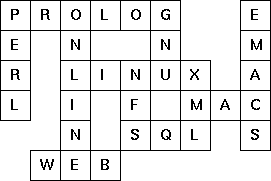Content of p99a.dat:

``````LINUX
PROLOG
PERL
ONLINE
GNU
XML
NFS
SQL
EMACS
WEB
MAC

......  .
. .  .  .
. ..... .
. . . ...
. ... .
...
``````

Words are strings of at least two characters. A horizontal or vertical sequence of character places in the crossword puzzle framework is called a site. Our problem is to find a compatible way of placing words onto sites.

### P100 (*****) Write a high-level language or at least DSL for SAT solvers.

E.g. something like Sentient Language 😄

• #### Moved to gradle kotlin dsl and did some cleanup

Tried simplifying stuff by:

• moving the tests to a standardized location (src/test/kotlin)
• removing the .idea folder
opened by uzilan 3

Corrected some typos and some suggests to make it flow better.. You asked me to do this when you first started this.. I've only got round to doing it now :(

I guess better late than never as such..

opened by chanshuikay 0

org.kotlin99.misc.SudokuDancingLinks.ExactCoverMatrix.Companion#toSudokuBoard does `it.header.toString().toInt()`. This should be refactored, e.g. to use Int as Node label.

opened by dkandalov 0
• #### Graph design

• overall `Graph` class seems a bit more complicated than you'd expect
• `Node` has `U` type parameter only to pass it into `Edge`
• there are too many methods in `Graph` companion object, ideally directed/undirected, labelled/unlabelled, terms/adjacency list should be "orthogonal"
• comparator in P84 https://github.com/dkandalov/kotlin-99/blob/8230bfa99c5bc692de9864d8ba0708dea6b041ff/src/org/kotlin99/graphs/P84.kt#L18-L18
opened by dkandalov 0
• #### Tree and Node design

1. Is it a good decision to model “no node" as `End` object? Or should it be `null` or may be a class?
2. What is the best way to model Nodes with XY position? I decided to wrap node value into object which has coordinates, unlike for example this solution http://aperiodic.net/phil/scala/s-99/p64.scala where they define `PositionedNode` class.
opened by dkandalov 0
###### Dmitry Kandalov###### Kotlin Examples Problems

Kotlin Examples Problems --->>> Repo: Getting Started Kotlin <<<--- --->>> Repo Kotlin Koans <<<--- --->>> Repo: GameBoy Emulator Enviroment <<<--- --

22 Oct 3, 2022
###### This repo contains my solutions to some data structures and algorithms problems on leetcode.

DSA Playground This repository contains solutions to dsa problems in kotlin. NOTE: This file will get long, please consider using <Ctrl>F DSA With Kun

2 Dec 9, 2021
###### Solutions to Hackerrank and CoderByte practice problems

Solutions to Hackerrank and CoderByte practice problems This repository contains solutions to CoderByte and Hackerrank practice problems with Kotlin.

2 Jul 5, 2022
###### Mocking for Kotlin/Native and Kotlin Multiplatform using the Kotlin Symbol Processing API (KSP)

Mockative Mocking for Kotlin/Native and Kotlin Multiplatform using the Kotlin Symbol Processing API (KSP). Installation Mockative uses KSP to generate

121 Dec 26, 2022
###### Kotlin-oop - Repositório criado para ser utilizado pelo projeto de Kotlin OOP desenvolvido em Kotlin nas aulas feitas através da plataforma Alura.

Projeto React OOP Repositório criado para ser utilizado pelo projeto de Kotlin OOP desenvolvido em Kotlin nas aulas feitas através da plataforma Alura

1 Jan 5, 2022
###### Kotlin-koans - Kotlin Koans are a series of exercises to get you familiar with the Kotlin Syntax

kotlin-koans-edu Kotlin Koans are a series of exercises to get you familiar with

1 Jan 11, 2022
###### Kotlin TodoMVC – full-stack Kotlin application demo

Kotlin full stack TodoMVC This project is an example implementation of the TodoMVC app written in Kotlin. More specifically, it's the Kotlin port of t

22 Oct 3, 2022
###### Integration Testing Kotlin Multiplatform Kata for Kotlin Developers. The main goal is to practice integration testing using Ktor and Ktor Client Mock

This kata is a Kotlin multiplatform version of the kata KataTODOApiClientKotlin of Karumi. We are here to practice integration testing using HTTP stub

29 Oct 3, 2022
###### Small kotlin library for persisting _single instances_ of kotlin data classes

PerSista Small library for persisting single instances of kotlin data classes. NB: PerSista uses typeOf() internally which is marked as @ExperimentalS

5 Nov 13, 2022
###### Kotlin Leaning Notes from Udacity Course | Kotlin Bootcamp for Programmers by Google

Kotlin Beginners Notes These are all personal notes taken from the Udacity Course (ud9011) of Kotlin Bootcamp for Programmers by Google as well as oth

34 Dec 10, 2022
###### Saga pattern implementation in Kotlin build in top of Kotlin's Coroutines.

Module Saga Website can be found here Add in build.gradle.kts repositories { mavenCentral() } dependencies { implementation("io.github.nomisr

50 Dec 30, 2022
###### Kotlin microservices with REST, and gRPC using BFF pattern. This repository contains backend services. Everything is dockerized and ready to "Go" actually "Kotlin" :-)

Microservices Kotlin gRPC Deployed in EC2, Check it out! This repo contains microservices written in Kotlin with BFF pattern for performing CRUD opera

18 Apr 21, 2022
###### A sample skeleton backend app built using Spring Boot kotlin, Expedia Kotlin Graphql, Reactive Web that can be deployed to Google App Engine Flexible environmennt

spring-kotlin-gql-gae This is a sample skeleton of a backend app that was built using: Spring Boot(Kotlin) Reactive Web Sprinng Data R2DBC with MYSQL

7 Sep 17, 2022
###### Modular Android architecture which showcase Kotlin, MVVM, Navigation, Hilt, Coroutines, Jetpack compose, Retrofit, Unit test and Kotlin Gradle DSL.

SampleCompose Modular Android architecture which showcase Kotlin, MVVM, Navigation, Hilt, Coroutines, Jetpack compose, Retrofit, Unit test and Kotlin

7 Nov 28, 2022
###### Learn-kotlin - Learning more about Kotlin in various content

Kotlin study roadmap https://kotlinlang.org/docs/reference/ Getting Started Basi

0 Jan 7, 2022
###### Mis experimentos con Kotlin para JetBrains Academy, certificación de Kotlin donde voy resolviendo proyectos de evaluación y haciendo actividades de cada tema.

Kotlin Academy Mis experimentos con Kotlin para JetBrains donde voy resolviendo proyectos de evaluación y haciendo actividades de cada tema. Acerca de

1 Jan 10, 2022
###### Repositório criado para ser utilizado pelo projeto de Kotlin Collections desenvolvido em Kotlin nas aulas feitas através da plataforma Alura.

Projeto Kotlin Collections Repositório criado para ser utilizado pelo projeto de Kotlin Collections desenvolvido em Kotlin nas aulas feitas através da

1 Jan 17, 2022
###### Kotlin-GraphQL-Apollo - Sencillo cliente para consumir una API GraphQL con Apollo usando Kotlin

Kotlin GraphQL Apollo Sencillo cliente para consumir una API GraphQL con Apollo

2 Jan 25, 2022
###### DS-for-Kotlin - Some classic data sturctures write in kotlin for fun

DS-for-Kotlin Just write some classic data structure by kotlin during my leisure

2 Jan 30, 2022